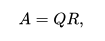﻿ NumPy: Calculate the QR decomposition of a given matrix - w3resourceNumPy: Calculate the QR decomposition of a given matrix

NumPy: Linear Algebra Exercise-13 with Solution

Write a NumPy program to calculate the QR decomposition of a given matrix.

From Wikipedia: In linear algebra, a QR decomposition (also called a QR factorization) of a matrix is a decomposition of a matrix A into a product A = QR of an orthogonal matrix Q and an upper triangular matrix R. QR decomposition is often used to solve the linear least squares problem and is the basis for a particular eigenvalue algorithm, the QR algorithm.

Square matrix
Any real square matrix A may be decomposed aswhere Q is an orthogonal matrix (its columns are orthogonal unit vectors meaning {\displaystyle Q^{\textsf {T}}Q=QQ^{\textsf {T}}=I} {\displaystyle Q^{\textsf {T}}Q=QQ^{\textsf {T}}=I}) and R is an upper triangular matrix (also called right triangular matrix). If A is invertible, then the factorization is unique if we require the diagonal elements of R to be positive.
If instead A is a complex square matrix, then there is a decomposition A = QR where Q is a unitary matrix (so {\displaystyle Q^{*}Q=QQ^{*}=I} {\displaystyle Q^{*}Q=QQ^{*}=I}).
If A has n linearly independent columns, then the first n columns of Q form an orthonormal basis for the column space of A. More generally, the first k columns of Q form an orthonormal basis for the span of the first k columns of A for any 1 ≤ k ≤ n. The fact that any column k of A only depends on the first k columns of Q is responsible for the triangular form of R.

Sample Solution :

Python Code :

import numpy as np
m = np.array([[1,2],[3,4]])
print("Original matrix:")
print(m)
result =  np.linalg.qr(m)
print("Decomposition of the said matrix:")
print(result)

Sample Output:

Original matrix:
[[1 2]
[3 4]]
Decomposition of the said matrix:
(array([[-0.31622777, -0.9486833 ],
[-0.9486833 ,  0.31622777]]), array([[-3.16227766, -4.42718872],
[ 0.        , -0.63245553]]))

Python Code Editor:

Have another way to solve this solution? Contribute your code (and comments) through Disqus.

What is the difficulty level of this exercise?

Test your Programming skills with w3resource's quiz.

﻿

Python: Tips of the Day

Time it:

timeit library is great for timing Python execution times. Just pass a function in string format to timeit.timeit() method and it will carry out 1 million executions to report the minimum time the code takes.

Its very useful to compare small code pieces and different functions but can be sluggish with big code.

Check out the example below demonstrating the execution time difference between 2 very similar list comprehension methods in Python:

import timeit
lst1='''list(range(100))'''
lst2='''[i for i in range(100)]'''
a=timeit.timeit(lst1)
b=timeit.timeit(lst2)
print(a, b, sep="------")

Output:

1.9301698543131351------5.389458132907748